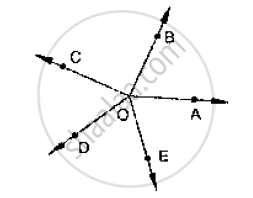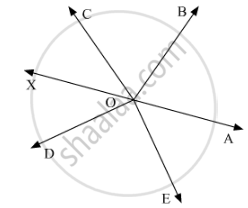# In the Given Below Fig, Rays Oa, Ob, Oc, Op and 0e Have the Common End Point O. Show that ∠Aob + ∠Boc + ∠Cod + ∠Doe + ∠Eoa = 360°. - Mathematics

In the given below fig, rays OA, OB, OC, OP and 0E have the common end point O. Show
that ∠AOB + ∠BOC + ∠COD + ∠DOE + ∠EOA = 360°.#### Solution 1

Given that

Rays OA, OB, OD and OE have the common end point O.

A ray of opposite to OA is drawn

Since ∠AOB, ∠BOF are linear pairs

∠AOB + ∠BOF = 180°

∠AOB + ∠BOC + ∠COF = 180°

Also

∠AOE, ∠EOF are linear pairs

∠AOE + ∠EOF = 180°

∠AOE + ∠DOF + ∠DOE = 180°

By adding (1) and (2) quations we get

∠AOB + ∠BOC + ∠COF + ∠AOE + ∠DOF + ∠DOE = 360°

∠AOB + ∠BOC + ∠COD + ∠DOE + ∠EOA = 360°

Hence proved.

#### Solution 2

Let us draw AOXa straight line.∠AOE,∠DOE and ∠DOXform a linear pair. Thus, their sum should be equal to180°.

Or, we can say that:

∠AOE +∠DOE +∠DOX  = 180°   (I)

Similarly,, ∠AOB,∠BOC and ∠COXform a linear pair. Thus, their sum should be equal to180°.

Or, we can say that:

∠AOB +∠BOC+ ∠COX =  180°       (II)

On adding (I) and (II), we get:

∠AOB +∠BOC + ∠COX +∠DOX +∠AOE +∠DOE = 180°+180°

∠AOB +∠BOC + ∠COD +∠AOE +∠DOE = 360°

Hence proved.

Concept: Introduction to Lines and Angles
Is there an error in this question or solution?

#### APPEARS IN

RD Sharma Mathematics for Class 9
Chapter 10 Lines and Angles
Exercise 10.2 | Q 4 | Page 14